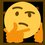# $\int { \sqrt { \tan { x } } } \, dx$

\begin{aligned} \int { \sqrt { \tan { x } } } \ dx & \overset { u\overset { let }{ = } \sqrt { \tan { x } } }{ = } \int { \frac { 2u^2 }{ { u }^{ 4 }+1 } du } \\ \quad & = \int { \frac { 2 }{ { u }^{ 2 }+\frac { 1 }{ { u }^{ 2 } } }du } \\ \quad & = \int { \frac { 1+\frac { 1 }{ { u }^{ 2 } } }{ { (u-\frac { 1 }{ u } ) }^{ 2 }+2 } du } +\int { \frac { 1-\frac { 1 }{ { u }^{ 2 } } }{ { (u+\frac { 1 }{ u } ) }^{ 2 }-2 } du } \\ \quad & \overset { \begin{matrix} v\overset { let }{ = } u-\frac { 1 }{ u } \\ w\overset { let }{ = } u+\frac { 1 }{ u } \end{matrix} }{ = } \int { \frac { 1 }{ { v }^{ 2 }+2 } dv } +\int { \frac { 1 }{ { w }^{ 2 }-2 } dw } \\ \quad & = \frac { 1 }{ \sqrt { 2 } } \tan ^{ -1 }{ \frac { v }{ \sqrt { 2 } } +{ c }_{ 1 } } -\frac { 1 }{ \sqrt { 2 } } \tanh ^{ -1 }{ \frac { w }{ \sqrt { 2 } } } +{ c }_{ 2 } \\ \quad & = \frac { \sqrt { 2 } }{ 2 } \tan ^{ -1 }{ \frac { (u-\frac { 1 }{ u } )\sqrt { 2 } }{ 2 } } -\frac { \sqrt { 2 } }{ 2 } \tanh ^{ -1 }{ \frac { (u+\frac { 1 }{ u } )\sqrt { 2 } }{ 2 } } +{ c } \\ \quad & = \boxed { \frac { \sqrt { 2 } }{ 2 } (\tan ^{ -1 }{ \frac { (\sqrt { \tan { x } } -\sqrt { \cot { x } } )\sqrt { 2 } }{ 2 } } -\tanh ^{ -1 }{ \frac { (\sqrt { \tan { x } } +\sqrt { \cot { x } } )\sqrt { 2 } }{ 2 } } )+c } \end{aligned}Note by Gandoff Tan
2 years, 2 months ago

This discussion board is a place to discuss our Daily Challenges and the math and science related to those challenges. Explanations are more than just a solution — they should explain the steps and thinking strategies that you used to obtain the solution. Comments should further the discussion of math and science.

When posting on Brilliant:

• Use the emojis to react to an explanation, whether you're congratulating a job well done , or just really confused .
• Ask specific questions about the challenge or the steps in somebody's explanation. Well-posed questions can add a lot to the discussion, but posting "I don't understand!" doesn't help anyone.
• Try to contribute something new to the discussion, whether it is an extension, generalization or other idea related to the challenge.

MarkdownAppears as
*italics* or _italics_ italics
**bold** or __bold__ bold
- bulleted- list
• bulleted
• list
1. numbered2. list
1. numbered
2. list
Note: you must add a full line of space before and after lists for them to show up correctly
paragraph 1paragraph 2

paragraph 1

paragraph 2

[example link](https://brilliant.org)example link
> This is a quote
This is a quote
    # I indented these lines
# 4 spaces, and now they show
# up as a code block.

print "hello world"
# I indented these lines
# 4 spaces, and now they show
# up as a code block.

print "hello world"
MathAppears as
Remember to wrap math in $$ ... $$ or $ ... $ to ensure proper formatting.
2 \times 3 $2 \times 3$
2^{34} $2^{34}$
a_{i-1} $a_{i-1}$
\frac{2}{3} $\frac{2}{3}$
\sqrt{2} $\sqrt{2}$
\sum_{i=1}^3 $\sum_{i=1}^3$
\sin \theta $\sin \theta$
\boxed{123} $\boxed{123}$

Sort by:

I finally found the answer to the hardest integral

- 1 year, 9 months ago

Wait, is tanh really involved?

- 2 years ago

Yes.

- 2 years ago